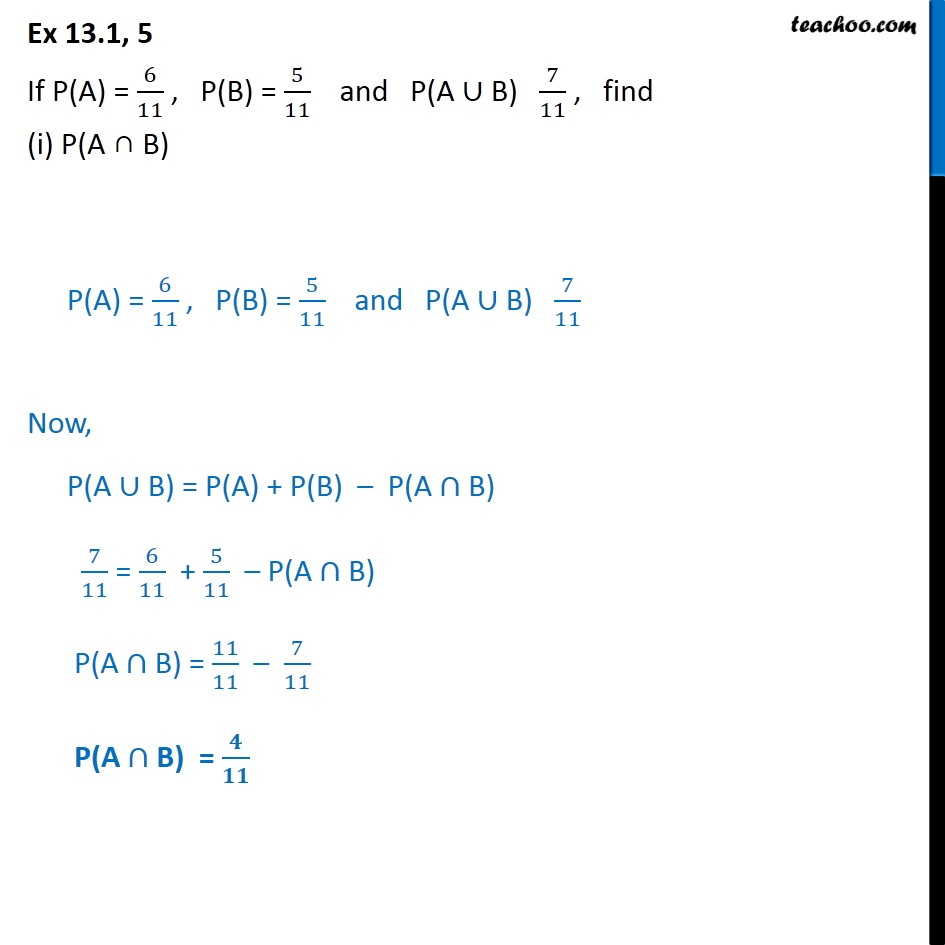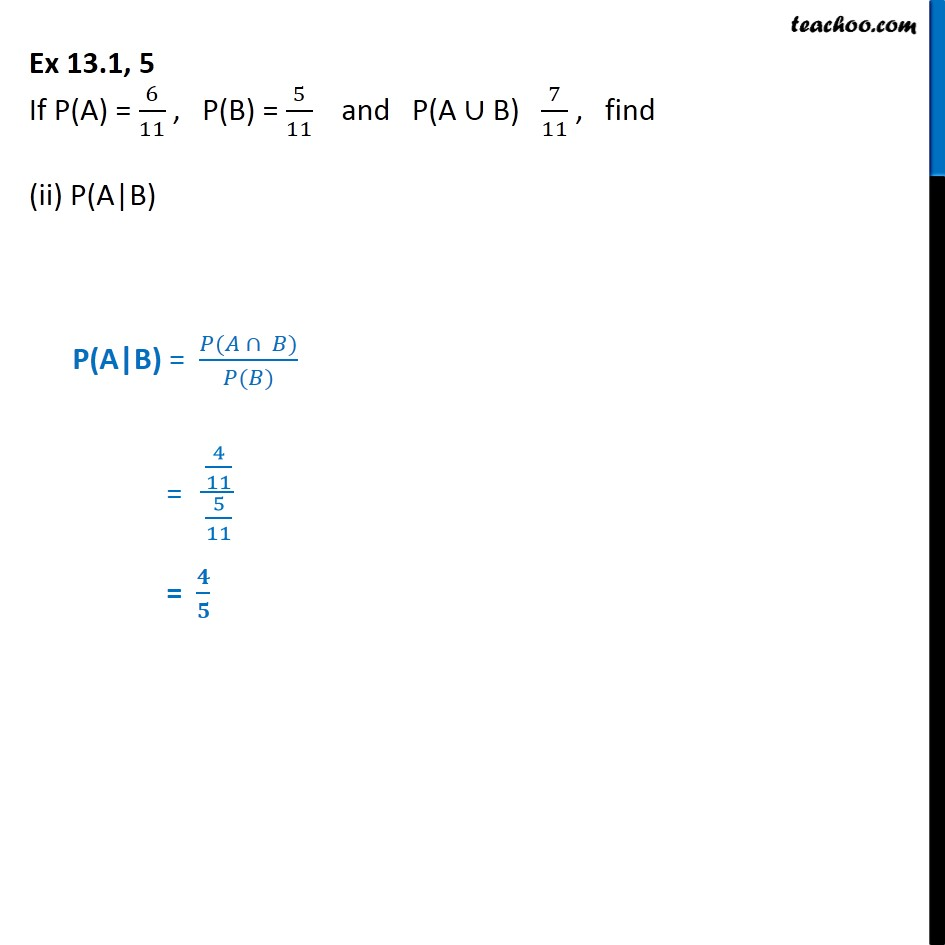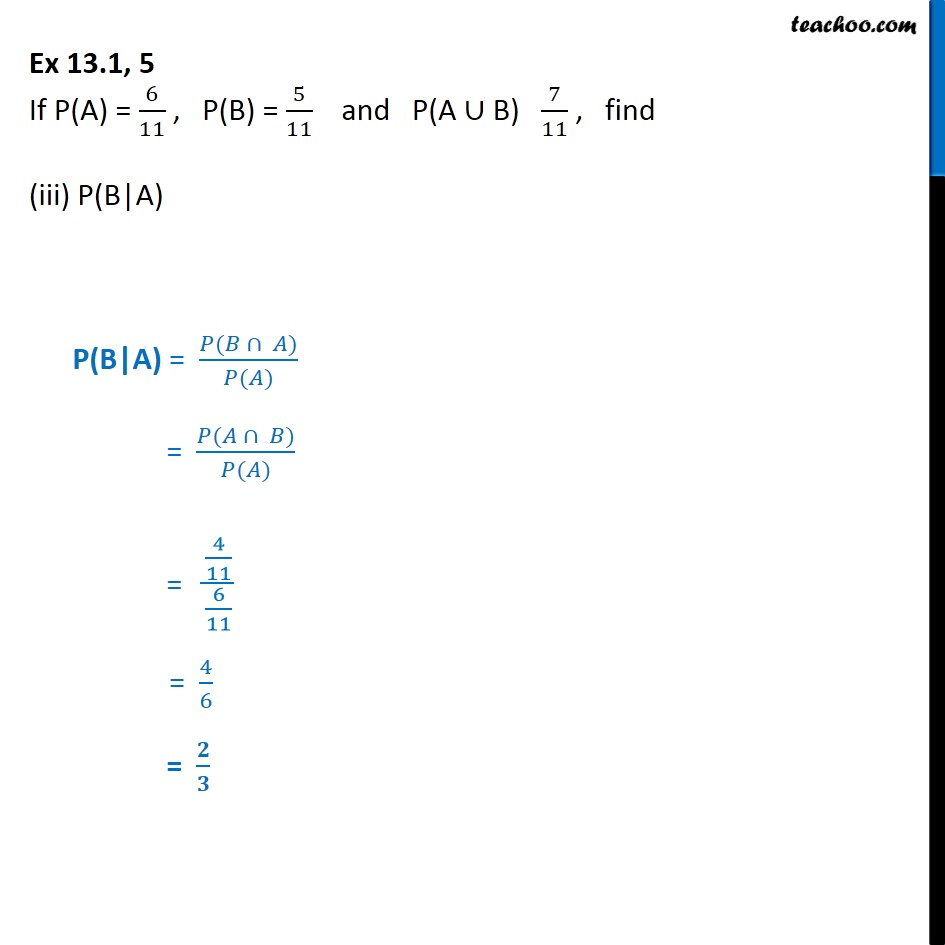1. Chapter 13 Class 12 Probability
2. Serial order wise
3. Ex 13.1

Transcript

Ex 13.1, 5 If P(A) = 6 11 , P(B) = 5 11 and P(A B) 7 11 , find (i) P(A B) P(A) = 6 11 , P(B) = 5 11 and P(A B) 7 11 Now, P(A B) = P(A) + P(B) P(A B) 7 11 = 6 11 + 5 11 P(A B) P(A B) = 11 11 7 11 P(A B) = Ex 13.1, 5 If P(A) = 6 11 , P(B) = 5 11 and P(A B) 7 11 , find (ii) P(A|B) P(A|B) = ( ) ( ) = 4 11 5 11 = Ex 13.1, 5 If P(A) = 6 11 , P(B) = 5 11 and P(A B) 7 11 , find (iii) P(B|A) P(B|A) = ( ) ( ) = ( ) ( ) = 4 11 6 11 = 4 6 =

Ex 13.1